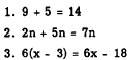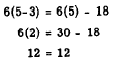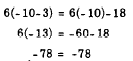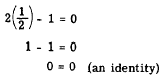Custom SearchDEGREE OF AN EQUATION The degree of an equation that has not more than one variable in each term is the exponent of the highest power to which that variable is raised in the equation. The equation     3x - 17=0 is a FIRST-DEGREE equation, since x is raised only to the first power. An example of a SECOND-DEGREE equation is         5x2 -2x+1=0. The equation,         4x3 - 7x2=0, is of the THIRD DEGREE. The equation, 3x - 2y = 5 is of the first degree in two variables, x and y. When more than one variable appears in a term, as in xy = 5, it is necessary to add the exponents of the variables within a term to get the degree of the equation. Since 1 + 1 = 2, the equation xy = 5 is of the second degree. LINEAR EQUATIONS Graphs are used in many different forms to give visual pictures of certain related facts. For example, they are used to show business trends, production output, continued individual attainment, and so forth. We find bar graphs, line graphs, circle graphs, and many other types, each of which is used for a particular need. In algebra, graphs are also used to give a visual picture containing a great .deal of information about equations. Sometimes many numerical values, when substituted for the variables of an equation, will satisfy the conditions of the equation. On a particular type of graph (which will be explained fully in chapter 12) several of these values are plotted (located), and when enough are plotted, a line is drawn through these points. For each particular equation a certain type of curve results. For equations in the first degree in one or two variables, the resulting shape of the "curve" is a straight line.. Thus, the name LINEAR EQUATION is derived. Equations of a higher degree form various other shapes. The name "linear equation" now applies to equations of the first degree, regardless of the number of variables they contain. Chapter 12 shows how an equation may be pictured on a graph. The purpose and value of graphing an equation will also be developed. IDENTITIES If a statement of equality involves one or more variables, it may be either an IDENTITY (identical equation) or a CONDITIONAL EQUATION. An identity is an equality that states a fact, such as the following examples:Notice that equation 3 merely shows the factored form of 6x - 18 and holds true when any value of x is substituted. For example, if x = 5, it becomesIf x assumes the negative value - 10, this identity becomesAn identity is established when both sides of the equality have been reduced to the same number or the same expression. When 5 is substituted for x, the value of either side of 6(x-3) = 6x - 18 is 12. When -10 is substituted for x, the value on either side is -78. The fact that this equality is an identity can be shown also by factoring the right side so that the equality becomes         6(x-3) = 6(x-3) The expressions on the two sides of the equality are identical. CONDITIONAL EQUATIONS A statement such as 2x - 1 = 0 is an equality only when x has one particular value. Such a statement is called a CONDITIONAL EQUATION, since it is true only under the condition that x = 1/2. Likewise, the equation y - 7 = 8 holds true only if y = 15. The value of the variable for which an equation in  one variable holds true is a ROOT, or SOLUTION, of the equation. When we speak of solving equations in algebra, we refer to conditional equations. The solution of a conditional equation can be verified by substituting for the variable its value, as determined by the solution. The solution. is correct if the equality reduces to an identity. For example, if 1/2 is substituted for x in 2x - 1 = 0, the result isThe identity is established for x = 1/2, since the value of each side of the equality reduces to zeroIntegrated Publishing, Inc. - A (SDVOSB) Service Disabled Veteran Owned Small Business